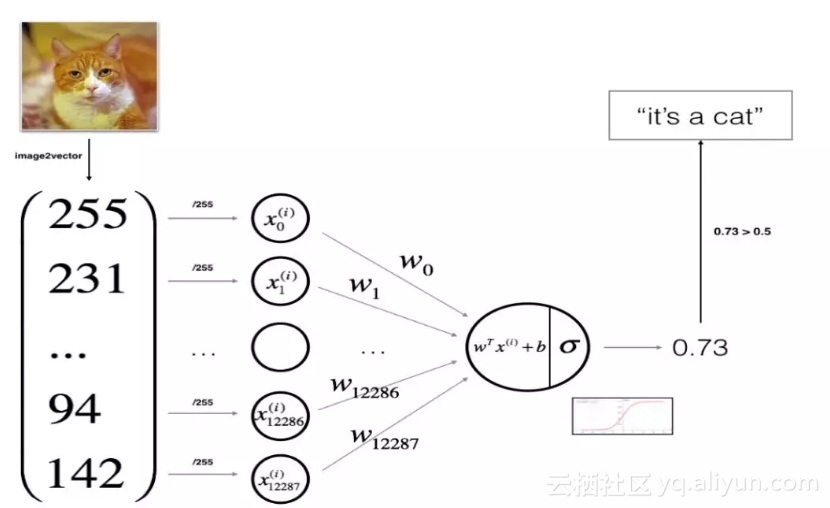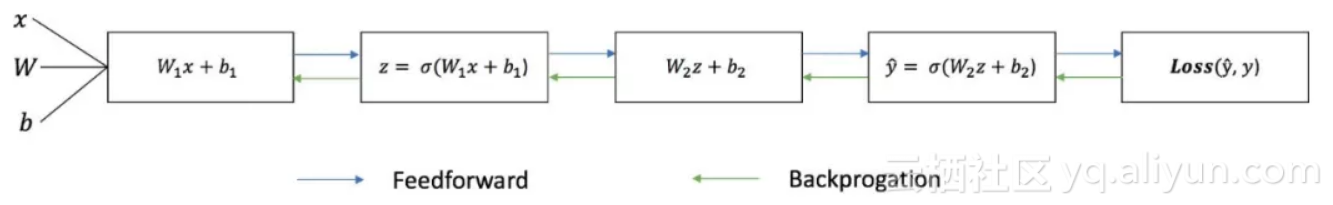# 深度学习笔记1：利用numpy从零搭建一个神经网络

+关注继续查看● 定义网络结构（指定输出层、隐藏层、输出层的大小）
● 初始化模型参数
● 循环操作：执行前向传播/计算损失/执行后向传播/权值更新

import numpy as np

def sigmoid(x):

return 1 / (1 + np.exp(-x))

def initilize_with_zeros(dim):

w = np.zeros((dim, 1))

b = 0.0 #assert(w.shape == (dim, 1)) #assert(isinstance(b, float) or isinstance(b, int)) return w, bdef propagate(w, b, X, Y):

m = X.shape

A = sigmoid(np.dot(w.T, X) + b)

cost = -1/m * np.sum(Y*np.log(A) + (1-Y)*np.log(1-A))

dw = np.dot(X, (A-Y).T)/m

db = np.sum(A-Y)/m

assert(dw.shape == w.shape)

assert(db.dtype == float)

cost = np.squeeze(cost)

assert(cost.shape == ())

'db': db

}

return grads, cost

def backward_propagation(w, b, X, Y, num_iterations, learning_rate, print_cost=False):

cost = []

for i in range(num_iterations):

grad, cost = propagate(w, b, X, Y)

w = w - learing_rate * dw

b = b - learning_rate * db

if i % 100 == 0:

cost.append(cost)

if print_cost and i % 100 == 0:

print("cost after iteration %i: %f" %(i, cost))

params = {"dw": w,

"db": b

}

"db": db

}

return params, grads, costs

def predict(w, b, X):

m = X.shape

Y_prediction = np.zeros((1, m))

w = w.reshape(X.shape, 1)

A = sigmoid(np.dot(w.T, X)+b)

for i in range(A.shape):

if A[:, i] > 0.5:

Y_prediction[:, i] = 1 else:

Y_prediction[:, i] = 0 assert(Y_prediction.shape == (1, m))

return Y_prediction

def model(X_train, Y_train, X_test, Y_test, num_iterations = 2000, learning_rate = 0.5, print_cost = False):

# initialize parameters with zeros (≈ 1 line of code)

w, b = initialize_with_zeros(X_train.shape) # Gradient descent (≈ 1 line of code)

parameters, grads, costs = backwize(w, b, X_train, Y_train, num_iterations, learning_rate, print_cost) # Retrieve parameters w and b from dictionary "parameters"

w = parameters["w"]

b = parameters["b"] # Predict test/train set examples (≈ 2 lines of code)

Y_prediction_train = predict(w, b, X_train)

Y_prediction_test = predict(w, b, X_test) # Print train/test Errors

print("train accuracy: {} %".format(100 - np.mean(np.abs(Y_prediction_train - Y_train)) * 100))

print("test accuracy: {} %".format(100 - np.mean(np.abs(Y_prediction_test - Y_test)) * 100))

d = {"costs": costs,

"Y_prediction_test": Y_prediction_test,

"Y_prediction_train" : Y_prediction_train,

"w" : w,

"b" : b,

"learning_rate" : learning_rate,

"num_iterations": num_iterations}

return dFTP（File Transfer Protocol，文件传输协议） 是 TCP/IP 协议组中的协议之一。在开发网站的时候，通常利用FTP协议把网页或程序传到Web服务器上。默认情况下FTP协议使用TCP端口中的 20和21这两个端口，其中20用于传输数据，21用于传输控制信息。
203 0Keras搭建深度神经网络解决多分类问题
2778 0【阿里云ACE】北京同城会 | 线下WORKSHOP 小白秒变大神搭建博客圆满完成

167 017251 0[网络摘录学习]常用的Linux系统监控命令
1318 07天ECS训练营学习笔记-如何在Linux环境下搭建ftp服务

250 0「72变的云开发」serverless搭建几乎零成本的typecho博客

267 02748 0

7195

9

+ 订阅

JS零基础入门教程（上册）